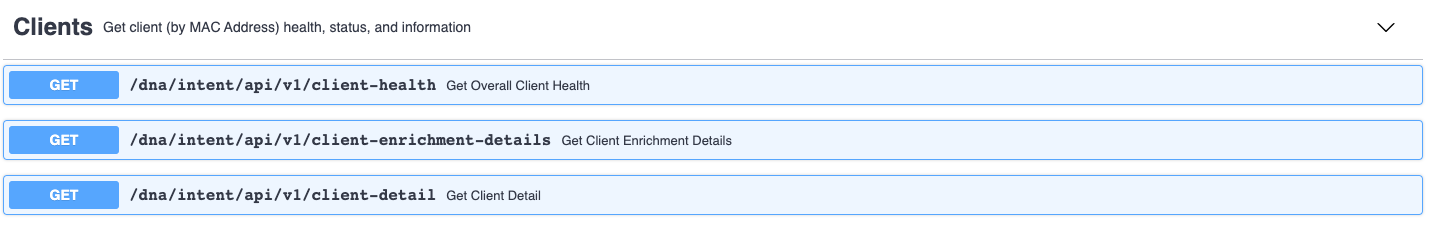# Cisco DNA Center - Assurance

### Introduction

In this post we introduced DNAC from a theoretical point of view. We continued in this post with looking at some simple Python scripts to retrieve device related information from DNAC. For this one, we will look into `Clients` API’s. We will write a Python script that shows us the health statistics per device category (wired or wireless).

### Get Client Health

Let’s have a look at the Client API section in the API documentation:As a reminder, we are looking to print an overview of the various health categories (fair, poor, …) per device category.

In below Python script, you will notice we use the `client-health` API. Before we dive into the Python code, let’s see how the response will look like.

``````wauterw@WAUTERW-M-65P7 Assurance % python3 get_client_health.py
DNAC: sandboxdnac2.cisco.com
{'response': [{'scoreDetail': [
{'clientCount': 66,
'clientUniqueCount': 66,
'endtime': 1587739500000,
'scoreCategory': {'scoreCategory': 'CLIENT_TYPE', 'value': 'ALL'},
'scoreValue': 36,
'starttime': 1587739200000},
{'clientCount': 2,
'clientUniqueCount': 2,
'endtime': 1587739500000,
'scoreCategory': {
'scoreCategory': 'CLIENT_TYPE','value': 'WIRED'},
'scoreList': [{'clientCount': 0,
'clientUniqueCount': 0,
'endtime': 1587739500000,
'scoreCategory': {
'scoreCategory': 'SCORE_TYPE','value': 'POOR'},

``````

So we’ll need to do some parsing to extract the data we are interested in.

Here’s what we do:

• We store the `ScoreDetails` in a list called `scores`
• We define two dictionaries, one for the wired devices and one for the wireless devices. We will populate those dictionaries later on.
• We iterate over the `scores` list and we look at the `value` of the `scoreCategory` key. This contains essentially whether the score is related to a wired device or a wireless device.
• Next, per category (wired or wireless) we will store `ScoreList` (which is an array) values in a list. As you can see, also the `ScoreList` has a `scoreCategory` itself. Now these scoreCategories are poor, fair, …. So we can now see
• We can then loop over the values and for each value (good, fair…) we add a key to the wired or wireless dictionary with the value (poor, fair…) and the clientCount as the corresponding value

The above might be a bit confusing I admit, but essentially all the above is done to get the following dictionary:

``````{
'wired':
{
'POOR': 0, 'FAIR': 0, 'GOOD': 2, 'IDLE': 0, 'NODATA': 0, 'NEW': 0
},
'wireless':
{
'POOR': 0, 'FAIR': 42, 'GOOD': 22, 'IDLE': 0, 'NODATA': 0, 'NEW': 0
}
}
``````

Note: this is in fact a dictionary inside another dictionary (cfr nested dictionaries).

With this dictionary, we have an elegant structure to show the health (as a percentage) for category. This is taken care of in the `calculatePercentageHealth()` function.

Here we do the following:

• We first calculate the total of client devices we have. We need this later on to be able to calculate the percentage.
• As we are dealing with a nested dictionary structure, we’ll need two for loops. The first loop gets us in either the wired or the wireless dictonary. The second loop will iterate over the inner dictionary and per category (poor, fair), calculate the client health as a percentage.
``````import requests
from authenticate import get_token
from pprint import pprint

def main():
dnac = "sandboxdnac2.cisco.com"
token = get_token(dnac)

url = f"https://{dnac}/dna/intent/api/v1/client-health"

"Content-Type": "application/json",
"Accept": "application/json",
"X-auth-Token": token
}

querystring = { "timestamp": ""}

scores = response['response']['scoreDetail']

d = {
'wired' : {},
'wireless': {}
}
nested_dict_wired = {}
nested_dict_wireless = {}

print("Overview")
print("--------")
for score in scores:
if score['scoreCategory']['value'] == 'ALL':
#print(f"Total devices - all: {score['clientCount']}")
print('')

if score['scoreCategory']['value'] == 'WIRED':
#print(f"  Total devices - wired: {score['clientCount']}")
values = score['scoreList']
for value in values:
#print(f"      {value['scoreCategory']['value']}: {value['clientCount']}")
nested_dict_wired[value['scoreCategory']['value']] = value['clientCount']
d['wired'] = nested_dict_wired

if score['scoreCategory']['value'] == 'WIRELESS':
#print(f"  Total devices - wireless: {score['clientCount']}")

values = score['scoreList']
for value in values:
#print(f"      {value['scoreCategory']['value']}: {value['clientCount']}")
nested_dict_wireless[value['scoreCategory']['value']] = value['clientCount']
d['wireless'] = nested_dict_wireless

calculatePercentageHealth(d)

def calculatePercentageHealth(d):
print("Percentage Health")
print("-----------------")
#Calculate Totals
sum_wired = 0
for key, value in d['wired'].items():
sum_wired += value

sum_wireless = 0
for key, value in d['wireless'].items():
sum_wireless += value

#Calculate Percentages
for key, value in d.items():
if key == 'wired':
print(f"Sum_wired: {sum_wired}")
for k, v in value.items():
percentage = round((v/sum_wired) * 100)
print(f"    For {k} => {percentage}%" )

if key == 'wireless':
print(f"Sum_wireless: {sum_wireless}")
for k, v in value.items():
percentage = round((v/sum_wireless) * 100)
print(f"    For {k} => {percentage}%" )

if __name__ == "__main__":
main()
``````

Executing this script, results in the following output:

``````Overview
--------

Percentage Health
-----------------
Sum_wired: 2
For POOR => 0%
For FAIR => 0%
For GOOD => 100%
For IDLE => 0%
For NODATA => 0%
For NEW => 0%
Sum_wireless: 64
For POOR => 0%
For FAIR => 66%
For GOOD => 34%
For IDLE => 0%
For NODATA => 0%
For NEW => 0%
``````

As usual, code can be found on my Github repo.